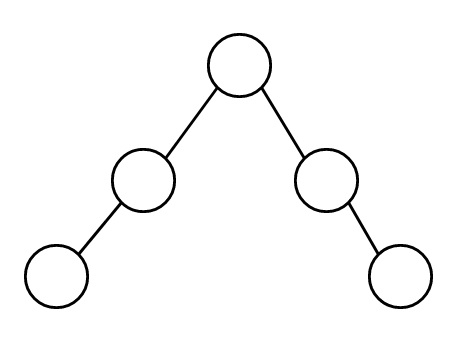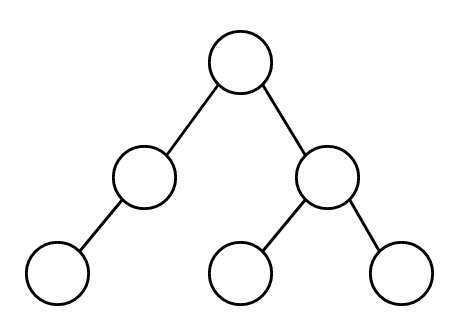# Check if a given tree graph is linear or not in C++

Here we will see how to check whether a tree graph is linear or not. A linear tree graph can be expressed in one line, suppose this is an example of a linear tree graph.But this is not linear −To check a graph is linear or not, we can follow two conditions

• If the number of nodes is 1, then the tree graph is linear
• If (n – 2) of its nodes have in-degree 2

## Example

Live Demo

#include <iostream>
#include <vector>
#define N 4
using namespace std;
class Graph{
private:
int V;
public:
Graph(int v){
V = v;
}
}
bool isLinear() {
if (V == 1)
return true;
int count = 0;
for (int i = 0; i < V; i++) {
count++;
}
if (count == V - 2)
return true;
else
return false;
}
};
int main() {
Graph g1(3);
}
The graph is linear